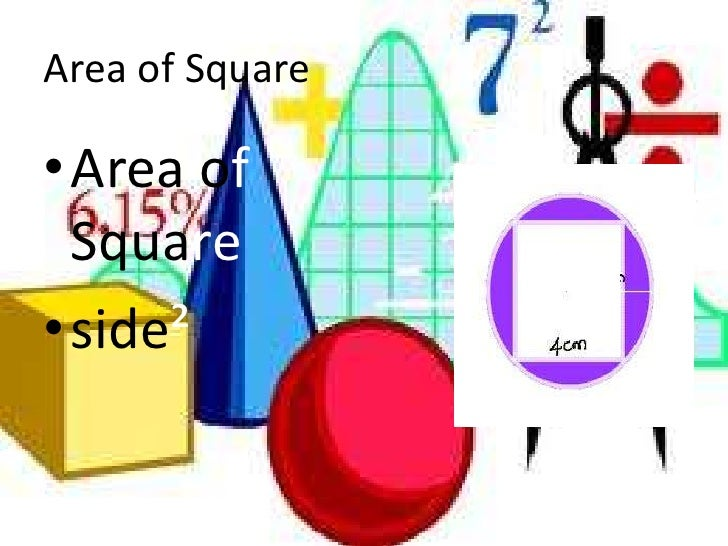# Quant Quiz on “MENSURATION” for SSC CGL 20161.       Find the volume(in cm^3) of a right circular cylinder of length 80 cm and diameter of the base 14 cm.
(a) 1400
(b) 1553
(c) 12320
(d) 13320

2.       The diameter of the base of a right circular cylinder is 14 cm, while its length is 40 cm. Find the total surface area of the cylinder.
(a) 2068 sq cm
(b) 1825 sq cm
(c) 1925 sq cm
(d) 2160 sq cm
3.     If the lateral surface area of a cylinder is 94.2 sq cm and its height is 5 cm, then find the radius of its base. (π = 3.14)
(a) 5 cm
(b) 8 cm
(c) 3 cm
(d) 4 cm
4.       A rod of 2 cm diameter and 30 cm length is converted into an electric  wire of 3 m length of uniform thickness. The diameter of the wire is:-
(a) 2/10 cm
(b) 2/√10  cm
(c) 1/√10 cm
(d) 1/10 cm
5.       What is the height of a solid cylinder of radius 5 cm and total surface area is 660 sq cm?
(a) 10 cm
(b) 12 cm
(c) 15 cm
(d) 16 cm
6.       What will be the curved surface area of a right circular cylinder having length 160 cm and radius of the base is 7 cm?
(a) 6020 sq cm
(b) 5052 sq cm
(c) 7045 sq cm
(d) 7040 sq cm
7.       The ratio of the radii of two cylinders is 2 : 3 and the ratio of their heights is 5 : 3. The ratio of their volumes will be:-
(a) 4 : 9
(b) 9 : 4
(c) 20 : 27
(d) 27 : 20
8.     The curved surface area of a cylindrical pillar is 264 sq m and its volume is 924 m^3 The ratio of its diameter to its height is:-
(a) 7 : 3
(b) 3 : 7
(c) 7 : 6
(d) 6 : 7
9.       If height of cylinder is decreased by 8%, while its radius remains unchanged, by what per cent does the volume decrease?
(a) 4%
(b) 6%
(c) 8%
(d) 10%
10.   If the radius of a cylinder is increased by 25% and its height remains unchanged, then find the per cent increase in volume.
(a) 56.25%
(b) 52.25%
(c) 50.4%
(d) 60.26%
11.   If the radius of cylinder is decreased by 8%, while its height is increased by 4%, what will be the effect on volume?
(a) 11.9744% (decrease)
(b) 11.9744% (increase)
(c) 12.4678% (decrease)
(d) 12.4678% (increase)
12.   A drainage tile is a cylindrical shell 21 cm long. The inside and outside diameters are 4.5 cm and 5.1 cm, respectively. What is the volume of the clay required for the tile?
(a) 6.96 π cm^3
(b) 6.76 π cm^3
(c) 5.76 π cm^3
(d) None of these

13.   The diameter of a road roller is 84 cm and its length 120 cm. It takes 500 complete revolutions to move once over to level a playground. Find the area of the playground (in sq m).
(a) 1632
(b) 1817
(c) 1532
(d) 1584

14.   In a temple a cylindrical pillar is 50 cm in diameter and 3.5 m in height. Find the cost of painting the curved surface of the piller at the rate of Rs. 10 per sq m.
(a) Rs. 50
(b) Rs. 68
(c) Rs. 98
(d) Rs. 55

15.   A Metro piller 14 cm in diameter is 5 m high. How much material was used to construct it?
(a)  (77×(10)^2 )  cm^3
(b) (77×(10)^4 )  cm^3
(c) (77×(10)^5 )  cm^3
(d) (77×(10)^3 )  cm^3

×

## Download success!

Thanks for downloading the guide. For similar guides, free study material, quizzes, videos and job alerts you can download the Adda247 app from play store.

Thank You, Your details have been submitted we will get back to you.

## Leave a comment

×
Login
OR

Forgot Password?

×
Sign Up
ORForgot Password
Enter the email address associated with your account, and we'll email you an OTP to verify it's you.Reset Password
Please enter the OTP sent to
/6

×
CHANGE PASSWORD